Subject: Estimating the shutoff head of a centrifugal pump S051-1

In the fifteenth century the Swiss scientist Daniel Bernoulli learned that the combination of head and velocity was a constant throughout a piping system. He then wrote the formula showing the relationship between this liquid velocity, and resultant head. As many of you know, I often quote this formula in my pump and seal schools. The formula looks like this: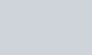V = Velocity or speed of the liquid at the impeller outside diameter (ft/sec. or meters/sec.)g = gravity = 32.2 feet / second2 or 9.8 meters / second2

My students have heard me quote this formula as the basis for my statement that you can estimate the shut off head of a 1750 rpm. centrifugal pump by squaring the diameter of the impeller. How did I come to that conclusion ? Lets look at the formula again, and we will start by defining velocity:

Velocity is a measurement of speed using distance and time as the variables. The terms we use to discuss velocity are feet/second or meters/ second. In the inch system the velocity of the impeller outside diameter is determined by the following formula: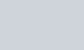d = diameter of the impellerrpm = speed of the impeller outside diameter12 = twelve inches in a foot60 sixty seconds in a minute

Now we will solve the formula. Substituting 1750 for the rpm we would get: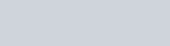Going back to the original formula we will substitute the new value for “V”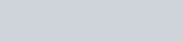This means that at 1750 rpm the shutoff head is 90% of the diameter of the impeller squared

If you will check a typical pump curve as supplied by the pump manufacturers, you will learn that the shut off head actually varies from 90% to 110% of the diameter of the impeller squared. I elected to use 100% because it is a sensible average and in some cases it accounts for the additional velocity added to the fluid as it moves from the impeller eye to the impeller outside diameter, or the loss when we have some internal recirculation.

If we substitute 3500 rpm for the speed, the new numbers would look like this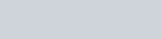Going back to the original formula we will substitute the new value for “V”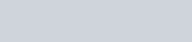We can round out the 3.6 to 4.0 and say that at 3500 rpm the shutoff head equals approximately the outside diameter of the impeller squared, times four.

It is a little trickier in the metric system. Instead of using millimeters when measuring the impeller diameter, move over two decimal places and use decimeters instead. It will make the calculations a lot simpler because you will be using more convenient, larger numbers.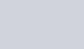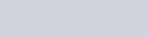Inserting the numbers into the formula we would get a velocity of: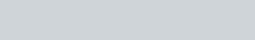Going back to the head formula we would get: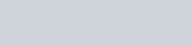We can round this off to 3d2

If the pump were running at 2900 rpm you would get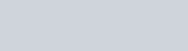Going back to the head formula we would get:We can round this off to 12d2

How do we use this information? You can combine this formula with your knowledge of how to convert pressure to head and come up with an estimate to see if an operating pump is operating close to its BEP(best efficiency point ). As an example:

In the inch system a pump discharge pressure gage reads 120 psi. The pump suction pressure gage reads 20 psi. The pump is pumping the difference between these readings, so the pump is pumping 100 psi.

At its BEP(best efficiency point) the pump should be running between 80% and 85% of its shut off head. 100 psi is 83% of 120 psi. The pressure to head conversion is: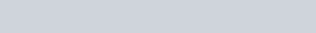The pump has an 8.5 inch impeller running at 3500 rpm. The shutoff head would be (8.5 inches)2 x 4 = 288 feet. Pretty close!

In the metric system we can make the calculation for a 295 millimeter impeller turning at 2900 rpm

The pump discharge pressure gage reads 10 bar The pump suction pressure gage reads 1 bar The pump is pumping the difference between these readings so the pump is pumping 9 bar

At its BEP(best efficiency point) the pump should be running between 80% and 85% of its shutoff head. 9 bar is 83% of 10.8 bar. The pressure to head conversion is: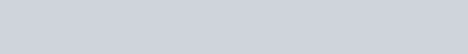106 meters shut off head. The pump has a 295 mm impeller running at 2900 rpm. The shutoff head would be (2.95 decimeter)2 x12 =104.4. Pretty close!

### Posted

• On February 17, 2018